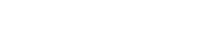# ISA Certified Control Systems Technician (CCST) program

• January 30, 2020
• CCST

Certified Control System Technicians (CCSTs) calibrate, document, troubleshoot, and repair/replace instrumentation for systems that measure and control level, temperature, pressure, flow, and other process variables.

### CCST question

If a flow sensor is connectied to the DCS system and the flow is displayed in volumetric units, what calculation would been to be performed in the control system to display the flow rate as a mass flow rate?

A. mass flow rate = volumetric flow rate × pipe cross-sectional area
B. mass flow rate = volumetric flow rate ×  sensor input in mA
C. mass flow rate = volumetric flow rate ×  fluid density
D. mass flow rate = volumetric flow rate / pipe cross-sectional area

The answer is C, mass flow rate = volumetric flow rate × fluid density.

To calculate mass flow rate, perform this calculation:

volumetric flow rate × fluid density

For example, using gallons for volume and LB for mass:

= gallons/min × LB/gallon

= LB/min

Reference: Goettsche, L.D. (Editor), Maintenance of Instruments and Systems, Second Edition, ISA, 2005.